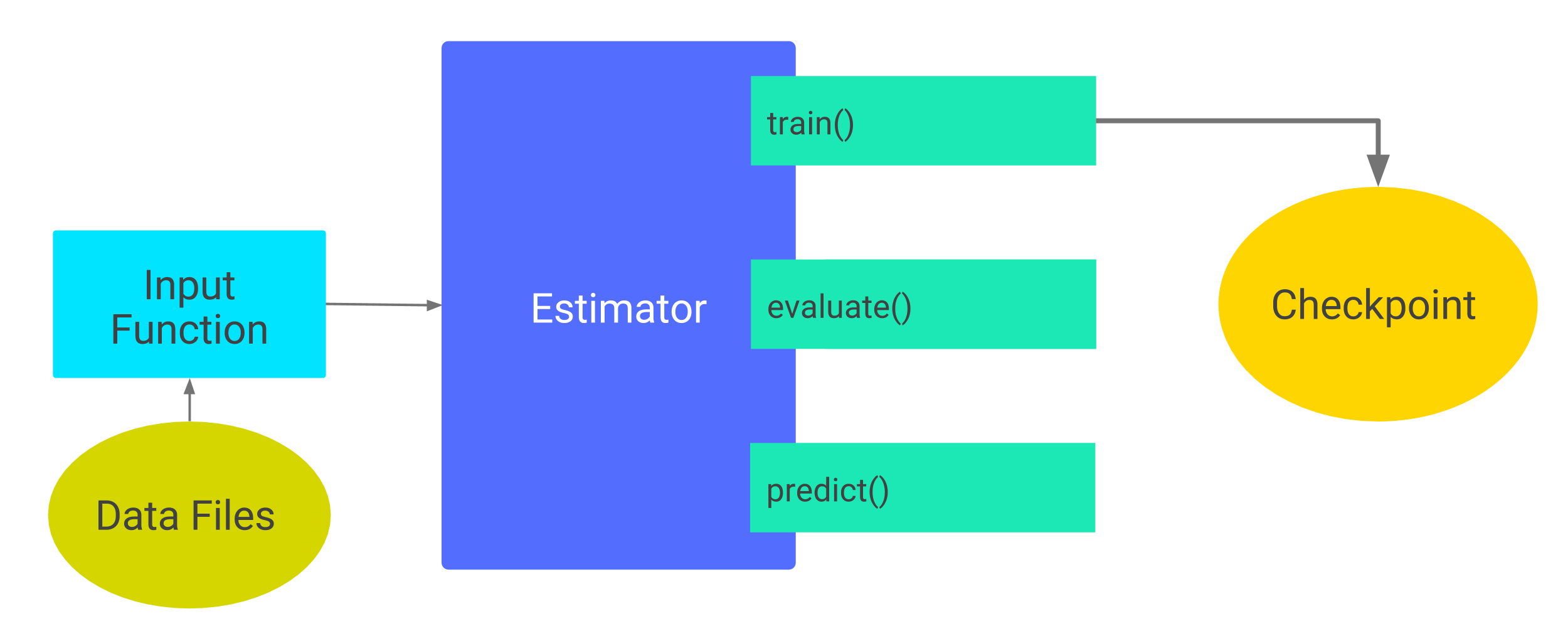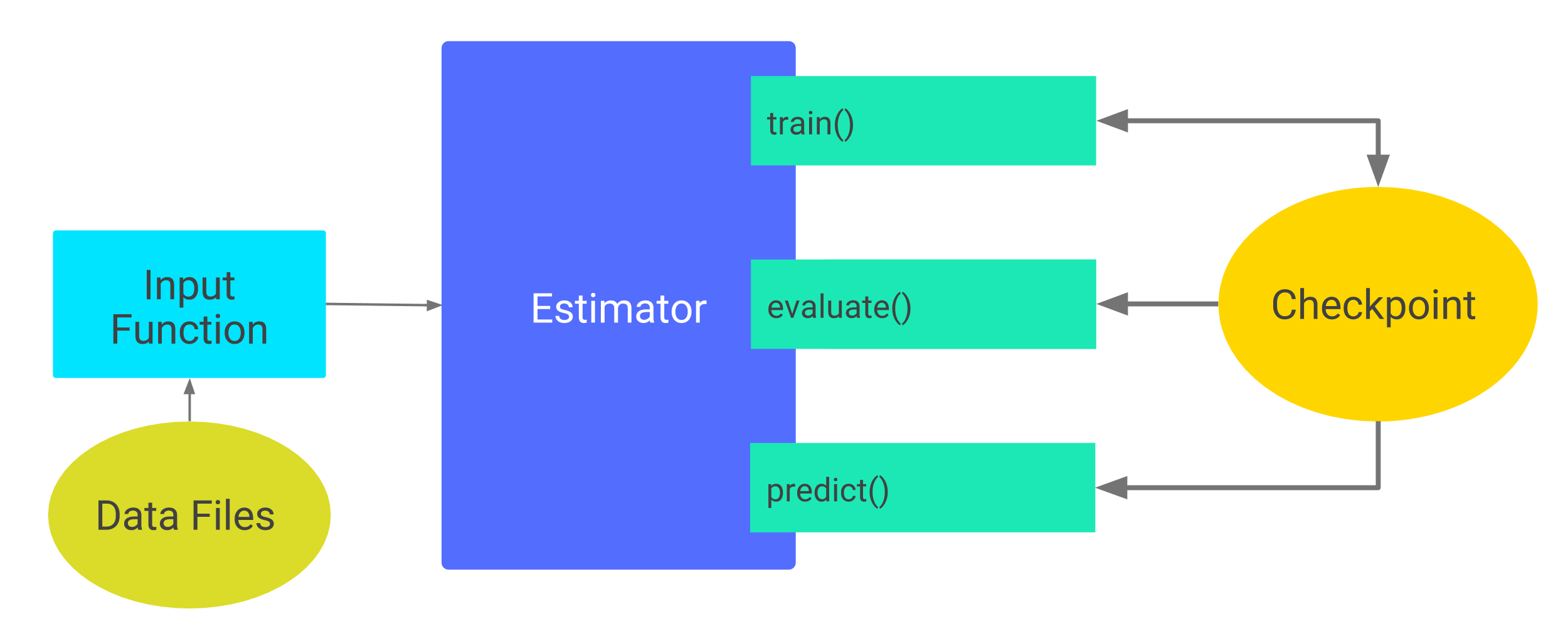TensorFlow 2.0 Beta is available

# Checkpoints

This document examines how to save and restore TensorFlow models built with Estimators. TensorFlow provides two model formats:

• checkpoints, which is a format dependent on the code that created the model.
• SavedModel, which is a format independent of the code that created the model.

This document focuses on checkpoints. For details on SavedModel, see the Saving and Restoring guide.

## Sample code

This document relies on the same Iris classification example detailed in Getting Started with TensorFlow. To download and access the example, invoke the following two commands:

git clone https://github.com/tensorflow/models/
cd models/samples/core/get_started


Most of the code snippets in this document are minor variations on premade_estimator.py.

## Saving partially-trained models

Estimators automatically write the following to disk:

• checkpoints, which are versions of the model created during training.
• event files, which contain information that TensorBoard uses to create visualizations.

To specify the top-level directory in which the Estimator stores its information, assign a value to the optional model_dir argument of any Estimator's constructor. Taking DNNClassifier as an example, the following code sets the model_dir argument to the models/iris directory:

classifier = tf.estimator.DNNClassifier(
feature_columns=my_feature_columns,
hidden_units=[10, 10],
n_classes=3,
model_dir='models/iris')


Suppose you call the Estimator's train method. For example:

classifier.train(
input_fn=lambda:train_input_fn(train_x, train_y, batch_size=100),
steps=200)


As suggested by the following diagrams, the first call to train adds checkpoints and other files to the model_dir directory:The first call to train().

To see the objects in the created model_dir directory on a UNIX-based system, just call ls as follows:

\$ ls -1 models/iris
checkpoint
events.out.tfevents.timestamp.hostname
graph.pbtxt
model.ckpt-1.data-00000-of-00001
model.ckpt-1.index
model.ckpt-1.meta
model.ckpt-200.data-00000-of-00001
model.ckpt-200.index
model.ckpt-200.meta


The preceding ls command shows that the Estimator created checkpoints at steps 1 (the start of training) and 200 (the end of training).

### Default checkpoint directory

If you don't specify model_dir in an Estimator's constructor, the Estimator writes checkpoint files to a temporary directory chosen by Python's tempfile.mkdtemp function. For example, the following Estimator constructor does not specify the model_dir argument:

classifier = tf.estimator.DNNClassifier(
feature_columns=my_feature_columns,
hidden_units=[10, 10],
n_classes=3)

print(classifier.model_dir)


The tempfile.mkdtemp function picks a secure, temporary directory appropriate for your operating system. For example, a typical temporary directory on macOS might be something like the following:

/var/folders/0s/5q9kfzfj3gx2knj0vj8p68yc00dhcr/T/tmpYm1Rwa


### Checkpointing Frequency

By default, the Estimator saves checkpoints in the model_dir according to the following schedule:

• Writes a checkpoint every 10 minutes (600 seconds).
• Writes a checkpoint when the train method starts (first iteration) and completes (final iteration).
• Retains only the 5 most recent checkpoints in the directory.

You may alter the default schedule by taking the following steps:

1. Create a tf.estimator.RunConfig object that defines the desired schedule.
2. When instantiating the Estimator, pass that RunConfig object to the Estimator's config argument.

For example, the following code changes the checkpointing schedule to every 20 minutes and retains the 10 most recent checkpoints:

my_checkpointing_config = tf.estimator.RunConfig(
save_checkpoints_secs = 20*60,  # Save checkpoints every 20 minutes.
keep_checkpoint_max = 10,       # Retain the 10 most recent checkpoints.
)

classifier = tf.estimator.DNNClassifier(
feature_columns=my_feature_columns,
hidden_units=[10, 10],
n_classes=3,
model_dir='models/iris',
config=my_checkpointing_config)


The first time you call an Estimator's train method, TensorFlow saves a checkpoint to the model_dir. Each subsequent call to the Estimator's train, evaluate, or predict method causes the following:

1. The Estimator builds the model's graph by running the model_fn(). (For details on the model_fn(), see Creating Custom Estimators.)
2. The Estimator initializes the weights of the new model from the data stored in the most recent checkpoint.

In other words, as the following illustration suggests, once checkpoints exist, TensorFlow rebuilds the model each time you call train(), evaluate(), or predict().Subsequent calls to train(), evaluate(), or predict()

Restoring a model's state from a checkpoint only works if the model and checkpoint are compatible. For example, suppose you trained a DNNClassifier Estimator containing two hidden layers, each having 10 nodes:

classifier = tf.estimator.DNNClassifier(
feature_columns=feature_columns,
hidden_units=[10, 10],
n_classes=3,
model_dir='models/iris')

classifier.train(
input_fn=lambda:train_input_fn(train_x, train_y, batch_size=100),
steps=200)


After training (and, therefore, after creating checkpoints in models/iris), imagine that you changed the number of neurons in each hidden layer from 10 to 20 and then attempted to retrain the model:

classifier2 = tf.estimator.DNNClassifier(
feature_columns=my_feature_columns,
hidden_units=[20, 20],  # Change the number of neurons in the model.
n_classes=3,
model_dir='models/iris')

classifier.train(
input_fn=lambda:train_input_fn(train_x, train_y, batch_size=100),
steps=200)


Since the state in the checkpoint is incompatible with the model described in classifier2, retraining fails with the following error:

...
InvalidArgumentError (see above for traceback): tensor_name =

To run experiments in which you train and compare slightly different versions of a model, save a copy of the code that created each model_dir, possibly by creating a separate git branch for each version. This separation will keep your checkpoints recoverable.